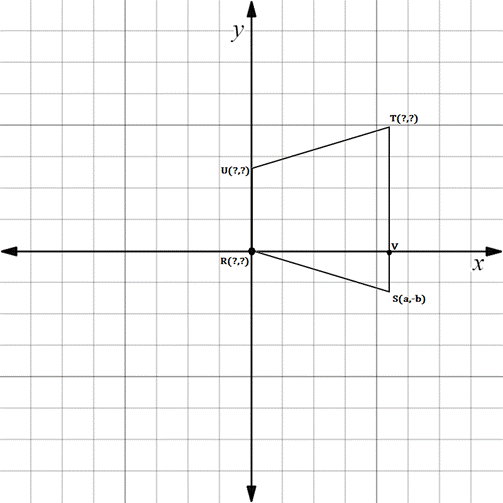Chapter 10.CR, Problem 29CRElementary Geometry For College St...

7th Edition
Alexander + 2 others
ISBN: 9781337614085

Solutions

Chapter
SectionElementary Geometry For College St...

7th Edition
Alexander + 2 others
ISBN: 9781337614085
Textbook Problem

In Review Exercise 27 to 30, supply the missing coordinates for the vertices, using as few variables as possible.Isosceles trapezoid R S T U with R V - ≅ R U -

To determine

To find:

The missing coordinates for the vertices.

Explanation

The given figure is shown below.

From the figure, the following observations are made.

Since, the vertex R is at the origin.

The coordinates of R0, 0.

In the problem, it is given that RV-RU-.

The horizontal distance from R to V is a.

Hence, the distance from R to U is also a.

That is, the y-coordinate of U is a.

In the vertex U, observe where it lies on the x-axis.

Since, it is at 0, the vertex U has the coordinates 0, a

Still sussing out bartleby?

Check out a sample textbook solution.

See a sample solution

The Solution to Your Study Problems

Bartleby provides explanations to thousands of textbook problems written by our experts, many with advanced degrees!

Get Started